table div table+table div table{width:100%;padding:0}table div table+table div table img{width:96.23%;padding:0;float:none}table div table+table div table td{width:100%;padding:0 1.88% 18px}/* styles */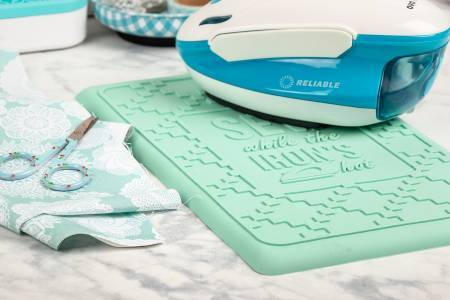This is one "hot" notion!

# New Goodies!

Rest your weary iron in between shots of steam and rounds of pressing! This silicone iron rest allows you to place your iron plate down without scorching your ironing surface, so you can “press on” without it tipping over. Fits most home irons and can withstand heat up to 500° F (260° C)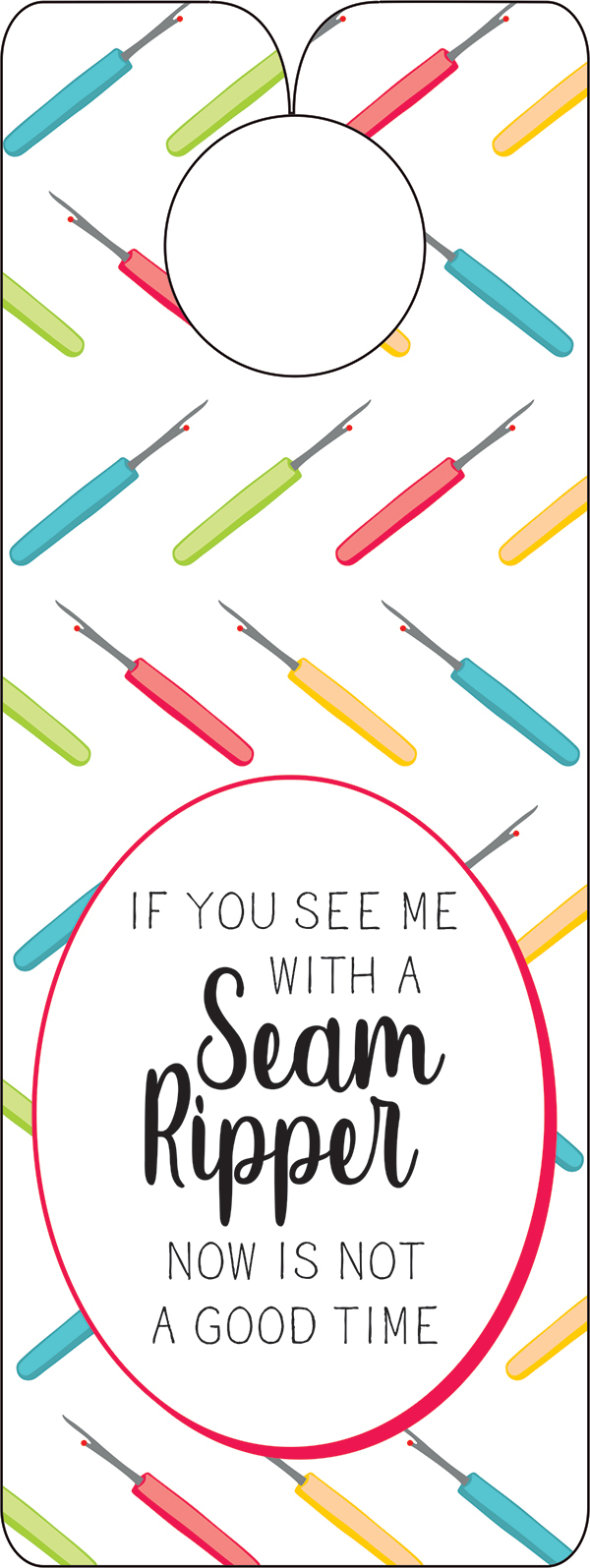Every quilting studio/closet/corner nook/etc needs a fun door hanger!
Click below to check out the 4 new ones!

 table div table+table+table+table+table+table div table{width:100%;padding:0}table div table+table+table+table+table+table div table img{width:96.23%;padding:0;float:none}table div table+table+table+table+table+table div table td{width:100%;padding:0 1.88% 18px}/* styles */## And, here's a little secret,

these brand new arrivals from Market were revealed for the first time on our Sunday Soiree, so if you like being first in-the-know, tune in on Sunday evenings at 7pm eastern time!

 table div table+table+table+table+table+table+table+table div table{width:100%;padding:0}table div table+table+table+table+table+table+table+table div table img{width:96.23%;padding:0;float:none}table div table+table+table+table+table+table+table+table div table td{width:100%;padding:0 1.88% 18px}/* styles */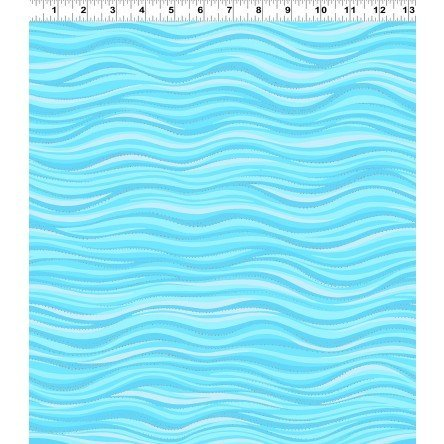# New Basic Fabrics

Laurel Burch fabrics with touches of metallic
Great yellow, perfect for Halloween moons and Christmas stars
The lightest, closest to white Batik I could find and a couple more!

 table div table+table+table+table+table+table+table+table+table+table+table div table{width:100%;padding:0}table div table+table+table+table+table+table+table+table+table+table+table div table img{width:96.23%;padding:0;float:none}table div table+table+table+table+table+table+table+table+table+table+table div table td{width:100%;padding:0 1.88% 18px}/* styles */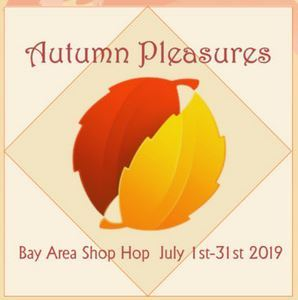# Autumn Pleasures Shop Hop

## Entire month of July!

Print out your passport and visit every shop during the month of July to register for prizes, purchase project kits, patterns and fabrics. What fun!

 table div table+table+table+table+table+table+table+table+table+table+table+table+table+table div table{width:100%;padding:0}table div table+table+table+table+table+table+table+table+table+table+table+table+table+table div table img{width:96.23%;padding:0;float:none}table div table+table+table+table+table+table+table+table+table+table+table+table+table+table div table td{width:100%;padding:0 1.88% 18px}/* styles */## Quilt-A-Holics Bus Tour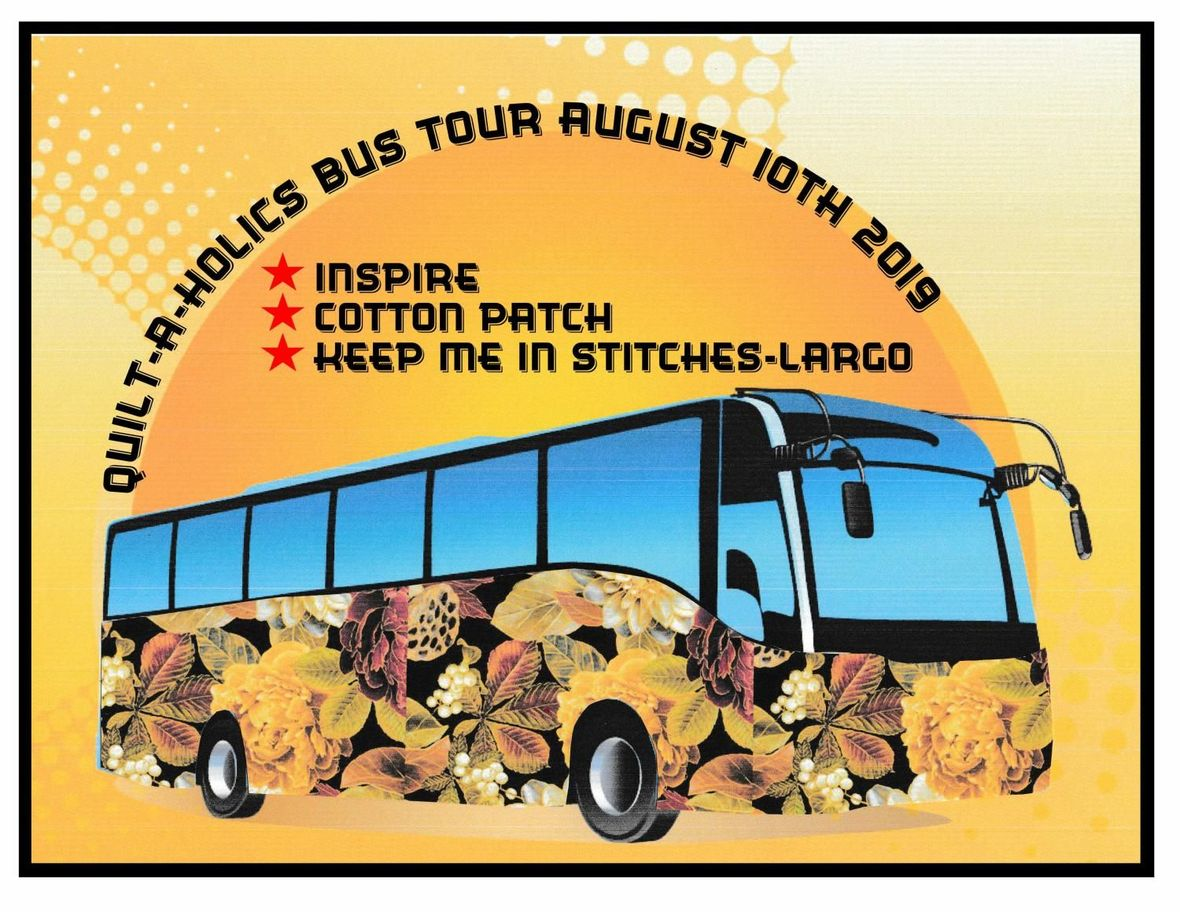# Saturday, August 10, 2019

## Stitches Largo - Cotton Patch

Join us for a fabulous day of quilt food, fabric and friends while someone else does the driving and takes care of lunch!

## Spots are going fast, don't wait!

 table div table+table+table+table+table+table+table+table+table+table+table+table+table+table+table+table+table+table div table{width:100%;padding:0}table div table+table+table+table+table+table+table+table+table+table+table+table+table+table+table+table+table+table div table img{width:96.23%;padding:0;float:none}table div table+table+table+table+table+table+table+table+table+table+table+table+table+table+table+table+table+table div table td{width:100%;padding:0 1.88% 18px}/* styles */# Next PMS Night is Friday, August 16

6pm to midnight
Treat yourself to a night of food, fabric and friends!
Who knows, you could be the next fat quarter winner!

 table div table+table+table+table+table+table+table+table+table+table+table+table+table+table+table+table+table+table+table+table+table div table{width:100%;padding:0}table div table+table+table+table+table+table+table+table+table+table+table+table+table+table+table+table+table+table+table+table+table div table img{width:96.23%;padding:0;float:none}table div table+table+table+table+table+table+table+table+table+table+table+table+table+table+table+table+table+table+table+table+table div table td{width:100%;padding:0 1.88% 18px}/* styles */# Casual Sew 10am to 4pm

Friday July 19, 26

# Upcoming Classes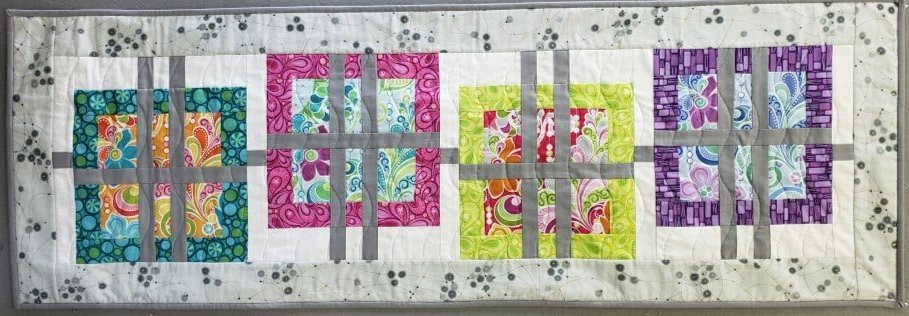# Woven Table Runner

Very fun runner - or it could be a quilt!
Class is Saturday, July 20

 table div table+table+table+table+table+table+table+table+table+table+table+table+table+table+table+table+table+table+table+table+table+table+table+table+table+table+table div table{width:100%;padding:0}table div table+table+table+table+table+table+table+table+table+table+table+table+table+table+table+table+table+table+table+table+table+table+table+table+table+table+table div table img{width:96.23%;padding:0;float:none}table div table+table+table+table+table+table+table+table+table+table+table+table+table+table+table+table+table+table+table+table+table+table+table+table+table+table+table div table td{width:100%;padding:0 1.88% 18px}/* styles */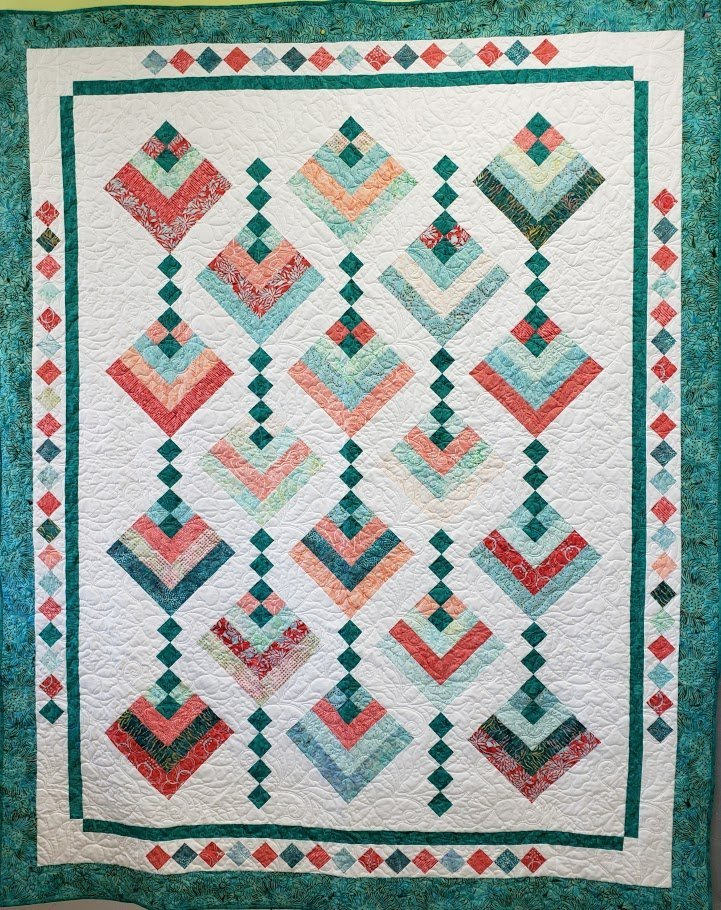# Hanging Garden

Hanging Gardens is a stunning quilt made from 2 ½” strips.
Marlyn will show you the tricks!
Class is Saturday, August 3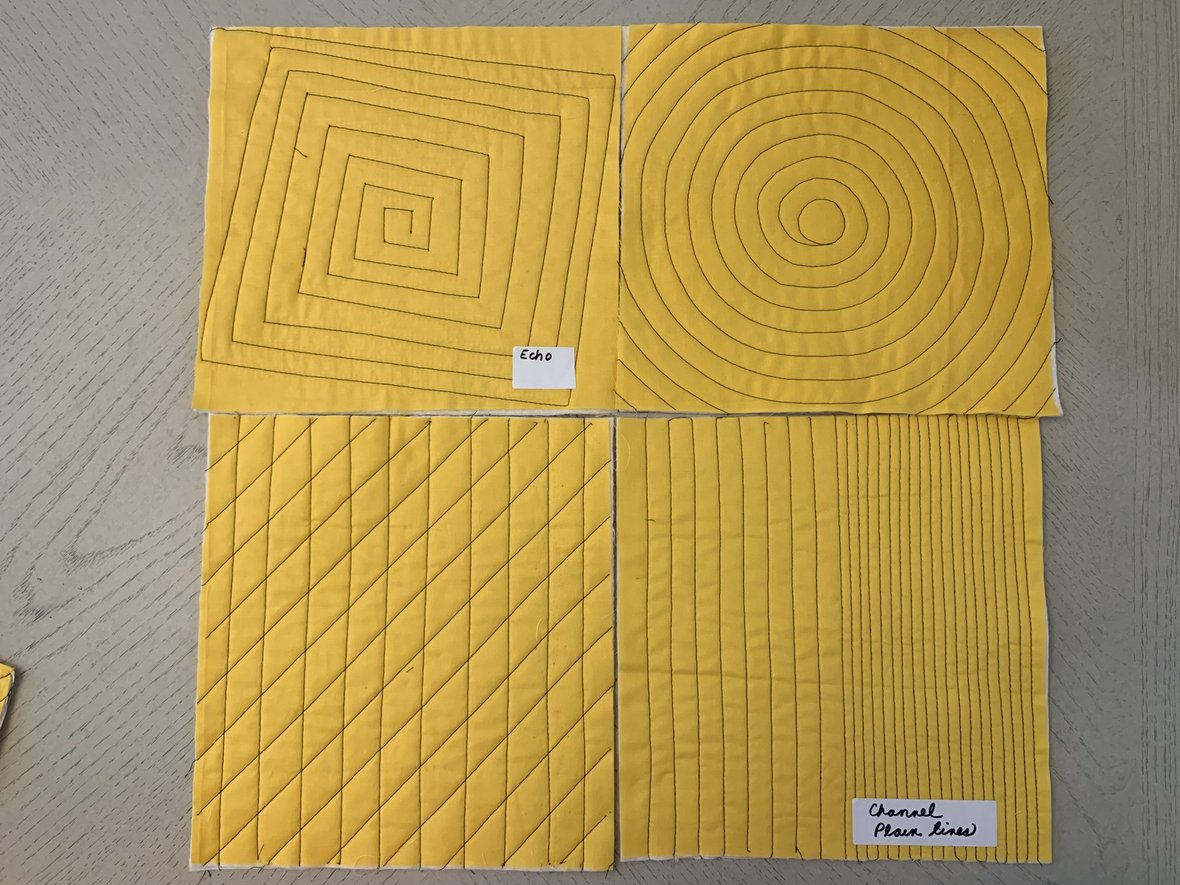# Walking Foot Wonders

Learn the basics of Straight-Line machine quilting.
This is a fun, quick, easy skill builder class, learning all the techniques.
Class is Monday, August 5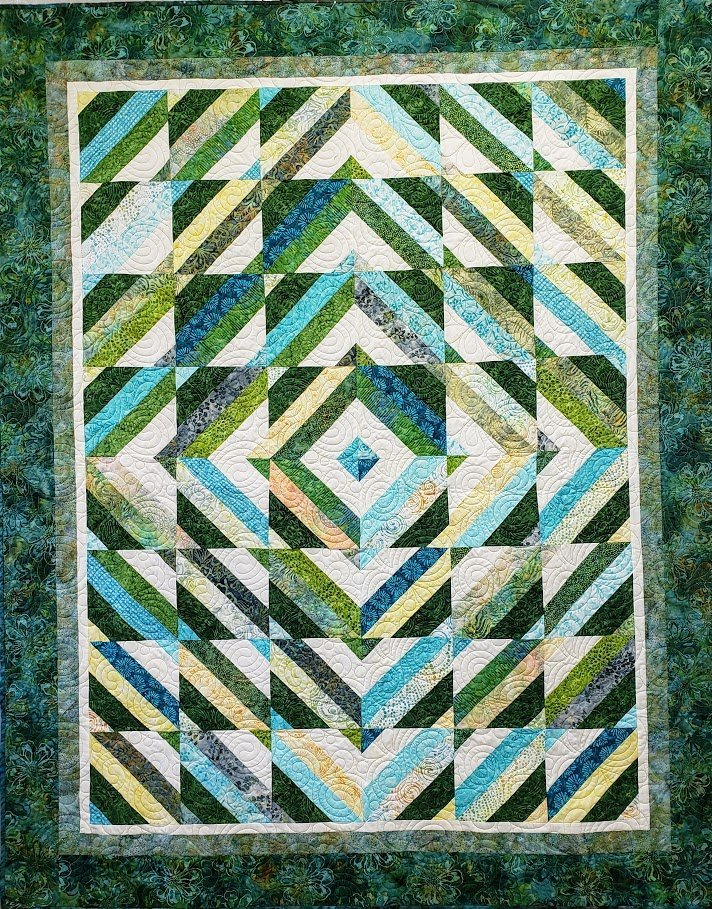# Tube Top

2 1/2" strips and a Strip Tube Ruler will make this quilt.
Take the class and Marlyn will share all her tips!
Class is Sunday August 11 and 18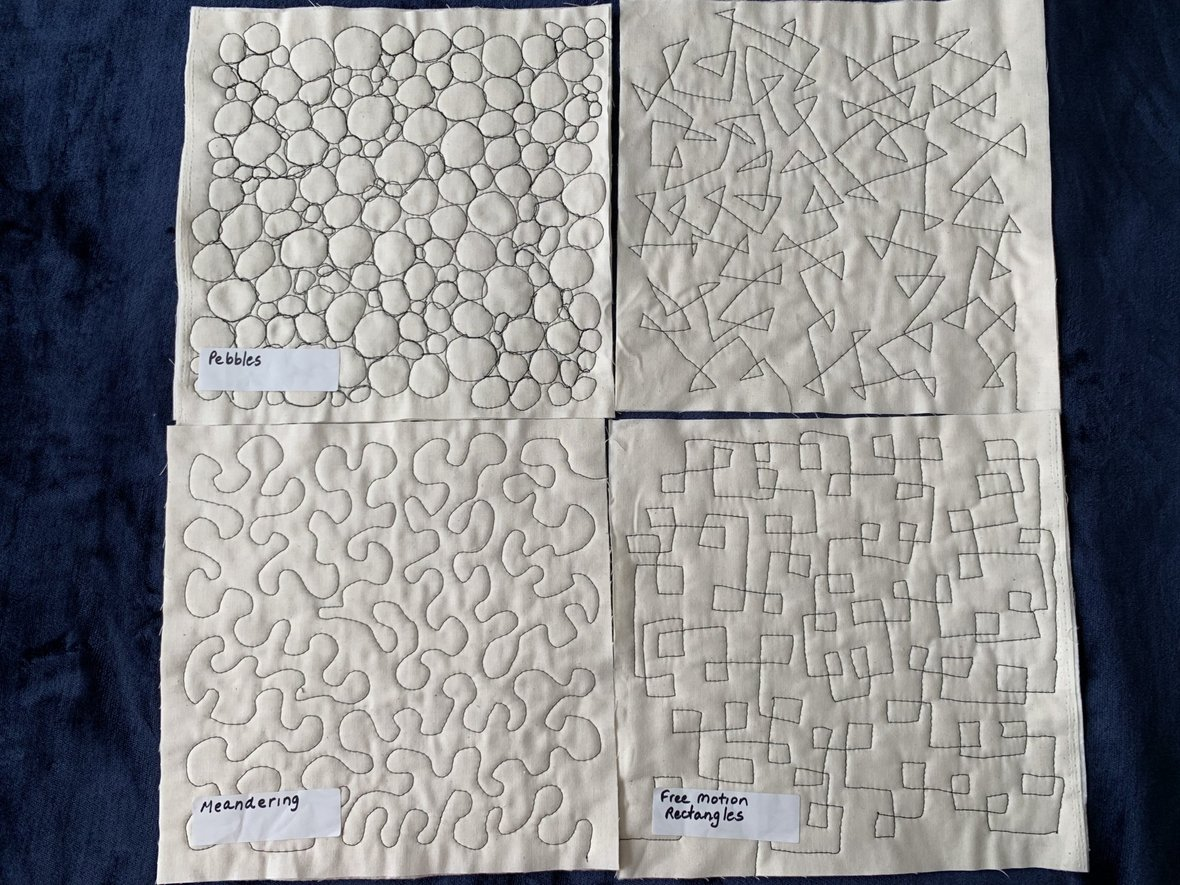# Free Motion Favorites

Learn the basics of Free Motion machine quilting.
This is a fun, quick, easy skill builder class, learning all the techniques.
Class is Monday, August 12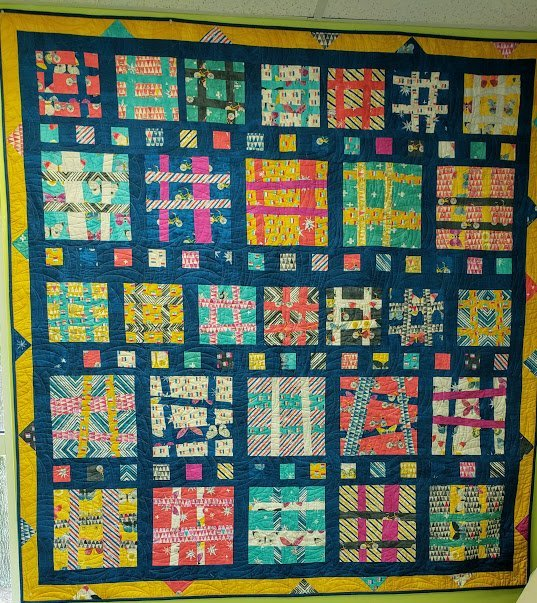Fun quilt to make, fun quilt to have!
Perfect to use a fat quarter bundle or dig into those coveted scraps!
Class is Wednesday, August 14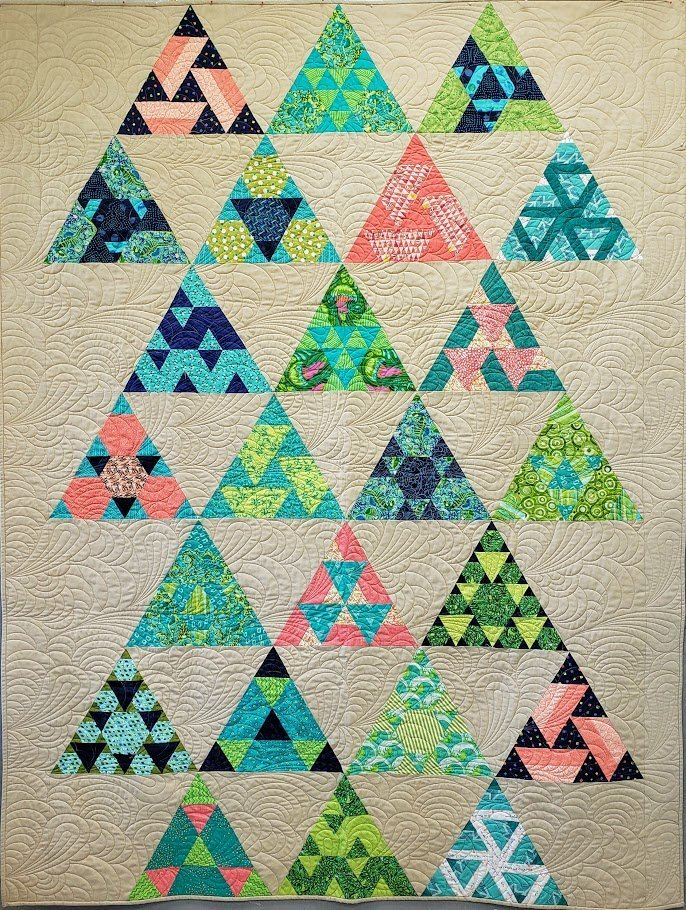# Toes in the Sand

Toes in the Sand is a block of the month quilt which we will be doing every three weeks.
Join in the fun and watch as you build this great quilt.
First session is Saturday, August 17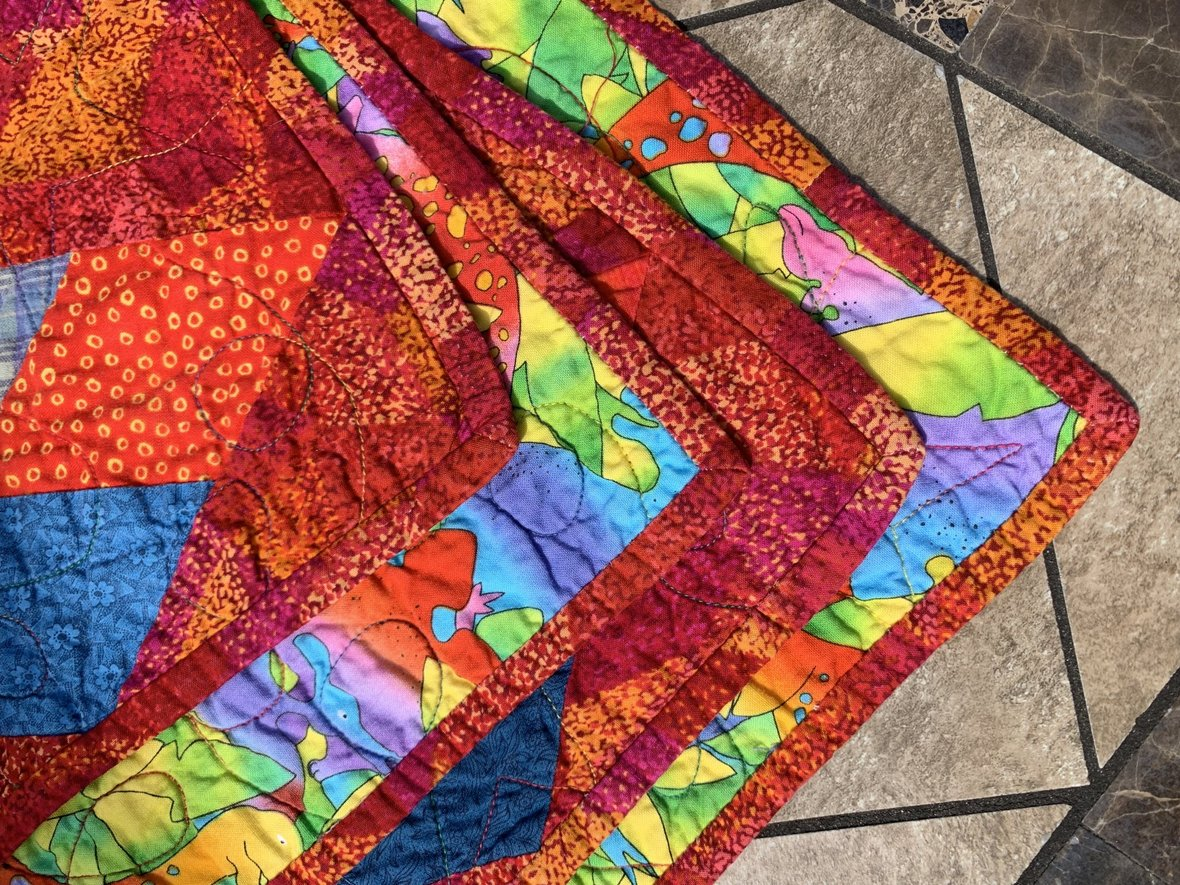# Brilliant Bindings

Learn the basics of finishing your quilt edges and applying professional looking binding.
This is a fun, quick, easy skill builder class, learning multiple techniques.
Class is Monday, August 19
and again Sunday, September 15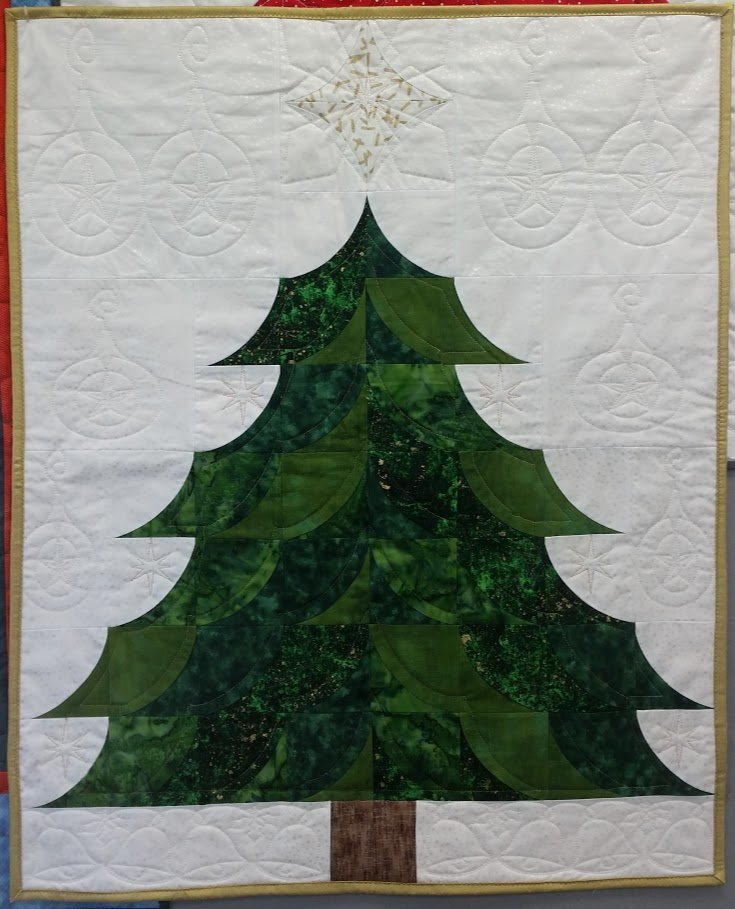# Giving Tree

Make a Christmas Tree wallhanging with the Mini Quick Curve Ruler!
Class is Tuesday, August 20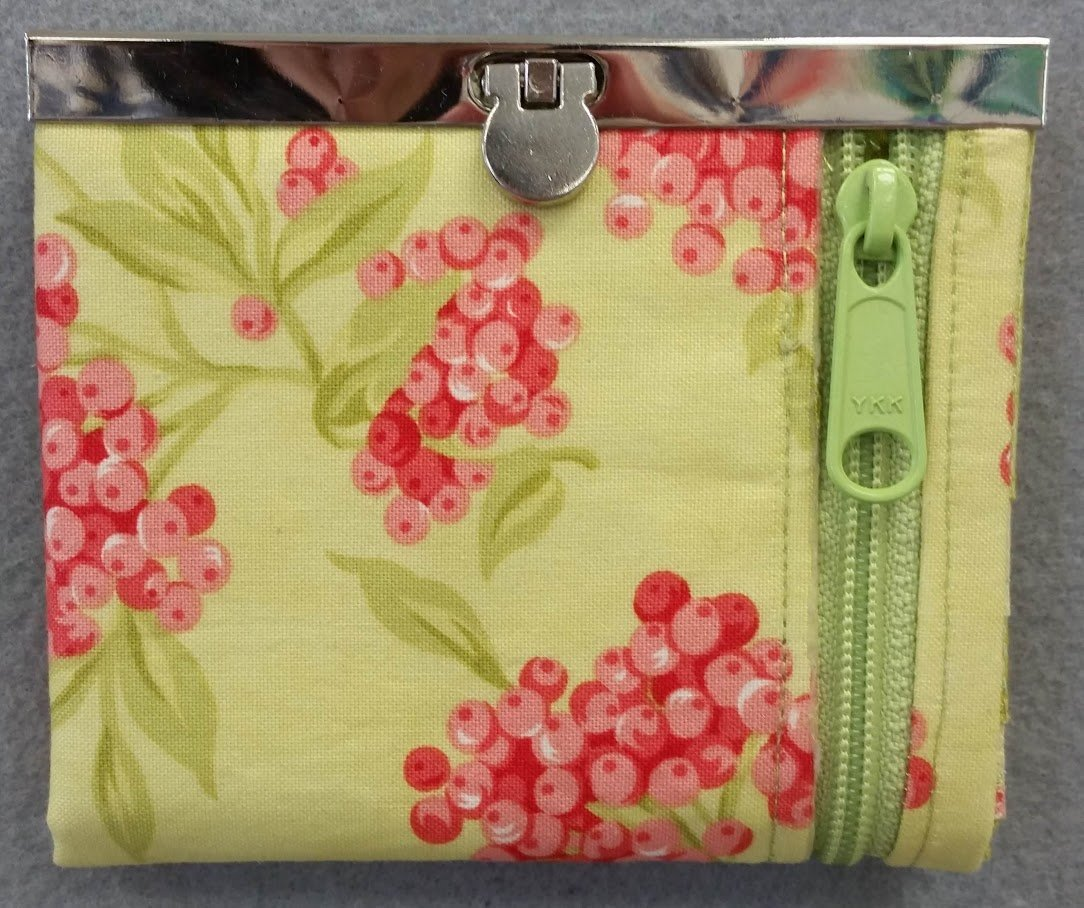# Mini Diva Wallet

Learn all the tricks to making this darling little wallet with a zipper and wallet frame.
Class is Saturday, August 24

# And there's more!

 table div table+table+table+table+table+table+table+table+table+table+table+table+table+table+table+table+table+table+table+table+table+table+table+table+table+table+table+table+table+table+table+table+table+table+table+table+table+table+table+table div table{width:100%;padding:0}table div table+table+table+table+table+table+table+table+table+table+table+table+table+table+table+table+table+table+table+table+table+table+table+table+table+table+table+table+table+table+table+table+table+table+table+table+table+table+table+table div table img{width:96.23%;padding:0;float:none}table div table+table+table+table+table+table+table+table+table+table+table+table+table+table+table+table+table+table+table+table+table+table+table+table+table+table+table+table+table+table+table+table+table+table+table+table+table+table+table+table div table td{width:100%;padding:0 1.88% 18px}/* styles */# FB Live is the place to be Sunday at 7pm!

You will be the 1st to see new fabrics, notions, kits and other great things that you need to know about!

There will be prizes, live demos and special pricing!

You will be able to purchase during the Live Broadcast and we can ship - sort of like Stuff Your Stocking but all in one night.

## If you miss the FB Live - not to worry!

You can view the replay on our FB Page AND you can still purchase at the special prices until 9pm Monday night!

We will go "live" at 6:55 and see what the hounds are up to, then
start the Soiree at 7pm (Eastern)

## Join me for a fun evening!

 table div table+table+table+table+table+table+table+table+table+table+table+table+table+table+table+table+table+table+table+table+table+table+table+table+table+table+table+table+table+table+table+table+table+table+table+table+table+table+table+table+table+table+table div table{width:100%;padding:0}table div table+table+table+table+table+table+table+table+table+table+table+table+table+table+table+table+table+table+table+table+table+table+table+table+table+table+table+table+table+table+table+table+table+table+table+table+table+table+table+table+table+table+table div table img{width:96.23%;padding:0;float:none}table div table+table+table+table+table+table+table+table+table+table+table+table+table+table+table+table+table+table+table+table+table+table+table+table+table+table+table+table+table+table+table+table+table+table+table+table+table+table+table+table+table+table+table div table td{width:100%;padding:0 1.88% 18px}/* styles */# Open 7 days a week for your convenience!

Monday through Saturday, 10am to 5pm
Sunday 12pm to 4pm

See you soon!

## Be sure to tune in to the Sunday Soiree on Facebook!

 table div table+table+table+table+table+table+table+table+table+table+table+table+table+table+table+table+table+table+table+table+table+table+table+table+table+table+table+table+table+table+table+table+table+table+table+table+table+table+table+table+table+table+table+table+table div table{width:100%;padding:0}table div table+table+table+table+table+table+table+table+table+table+table+table+table+table+table+table+table+table+table+table+table+table+table+table+table+table+table+table+table+table+table+table+table+table+table+table+table+table+table+table+table+table+table+table+table div table img{width:96.23%;padding:0;float:none}table div table+table+table+table+table+table+table+table+table+table+table+table+table+table+table+table+table+table+table+table+table+table+table+table+table+table+table+table+table+table+table+table+table+table+table+table+table+table+table+table+table+table+table+table+table div table td{width:100%;padding:0 1.88% 18px}/* styles */